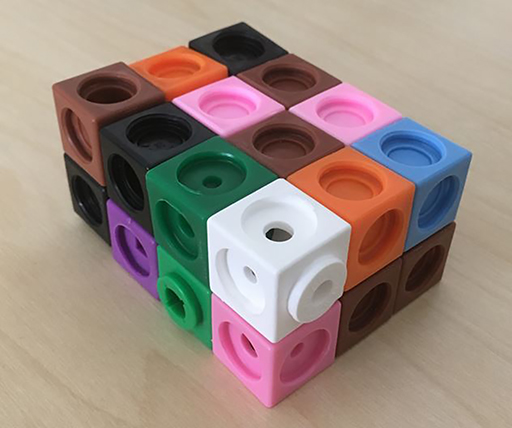Teaching mathematics

Start this free course now. Just create an account and sign in. Enrol and complete the course for a free statement of participation or digital badge if available.

Free course

# 4.3 Volume

Volume is a measurement of the amount of space inside a 3D shape and is reckoned by the number of unit cubes inside the shape. Common metric units of volume are cubic centimetres (cm3) or cc, and cubic metres (m3). Examples of imperial measures of volume are cubic inches and cubic feet.

It is best to begin the topic of volume by considering the inside of 3D shapes as being divided into unit cubes with volume being recorded as the number of cubes and the units of volume (e.g. 12 cm3). Volume can be investigated practically using 1 × 1 × 1 cm multilink cubes.

For example, learners can be asked to use multilink cubes to make a cuboid which is 3 cm by 4 cm by 2 cm and to work out how many cubes are needed to make it (Figure 14).

Investigating different sized cuboids should lead to the formula for the volume of a cuboid as

Volume = length × width × height.

As with the area formulas, the volume of other more complicated 3D shapes can be derived from the formula of the volume of a cuboid.Figure 14 A 4 × 3 × 2 cuboid made of 24 unit cubes
TM_1